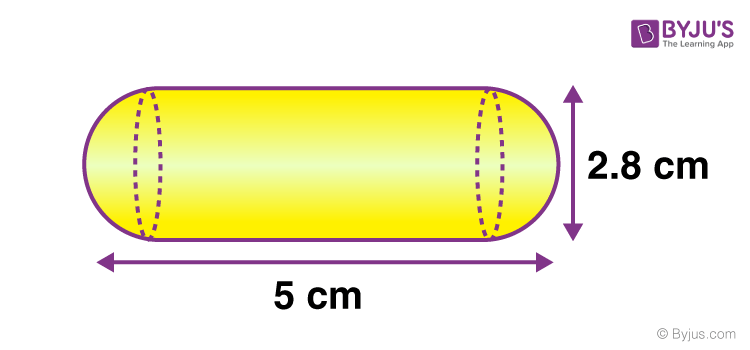# A gulab jamun contains sugar syrup up to about 30% of its volume. Find approximately how much syrup should be found in 45 gulab jamuns, each shaped like a cylinder with two hemispherical ends with a length of 5 cm and diameter of 2.8 cm as shown in the below figure.Given parameters:

Each gulab jamun is given the form of a cylinder with two hemispherical ends.

Diameter of the cylinder = 2.8 cm

Radius of the cylinder = Diameter of cylinder/2

Diameter of the hemisphere = 2.8 cm

Radius of the hemisphere = Diameter of hemisphere/2

Radius of the hemisphere (r) = 2.8 /2

r = 1.4 cm

Height of the cylinder + 2 ✕ Radius of the hemisphere = 5 cm

Height of the cylinder = 5 – (2 ✕ Radius of the hemisphere)

Height of the cylinder = 5 – (2 ✕ 1.4)

Height of the cylinder (h) = 2.2 cm

Volume of Cylinder = πr2h

Volume of cylinder = (22/7) ✕ (1.4)2 ✕ 2.2

Volume of cylinder = 13.552 cm3

Volume of hemisphere = (2/3) πr3

Volume of hemisphere = (2/3) ✕ (22/7) ✕ (1.4)3

Volume of hemisphere = 0.667 ✕ 3.143 ✕ 2.744

Volume of hemisphere = 5.75 cm3

Now

Volume of two hemisphere = 2 ✕ 5.75 cm3

Volume of two hemisphere = 11.5 cm3

Let us calculate the volume of the gulab jamun

Volume of gulab jamun = Volume of the cylinder + Volume of two hemisphere

Volume of gulab jamun = 13.552 cm3 + 11.5 cm3

Volume of gulab jamun = 25.052 cm3

The volume of one gulab jamun is 25.052 cm3

∴ The volume of 45 gulab jamun = 25.052 cm3 ✕ 45

The volume of 45 gulab jamun = 1127.34 cm3

In gulab jamun quantity of sugar syrup = 30% = 30/100 = 0.3

The quantity of sugar syrup in 45 gulab jamun = 0.3 ✕ volume of one gulab jamun ✕ 45

The quantity of sugar syrup in 45 gulab jamun = 0.3 ✕ 25.052 ✕ 45

The quantity of sugar syrup in 45 gulab jamun = 338.25 cm3.(105)(35)